Instant Maths Help:

# Edexcel A Level - Algebraic Expressions

## Simplifying Algebraic Expressions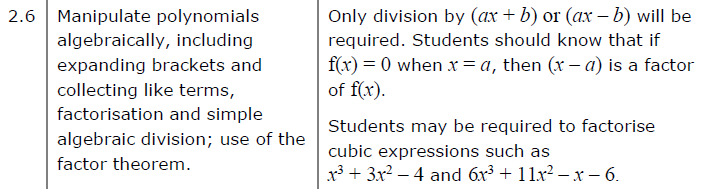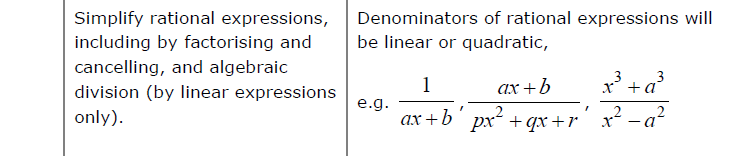##### Edexcel 9MA0/01 Jun 2023 A2 Exam Q. 2 :   6 marks in 7:12 min.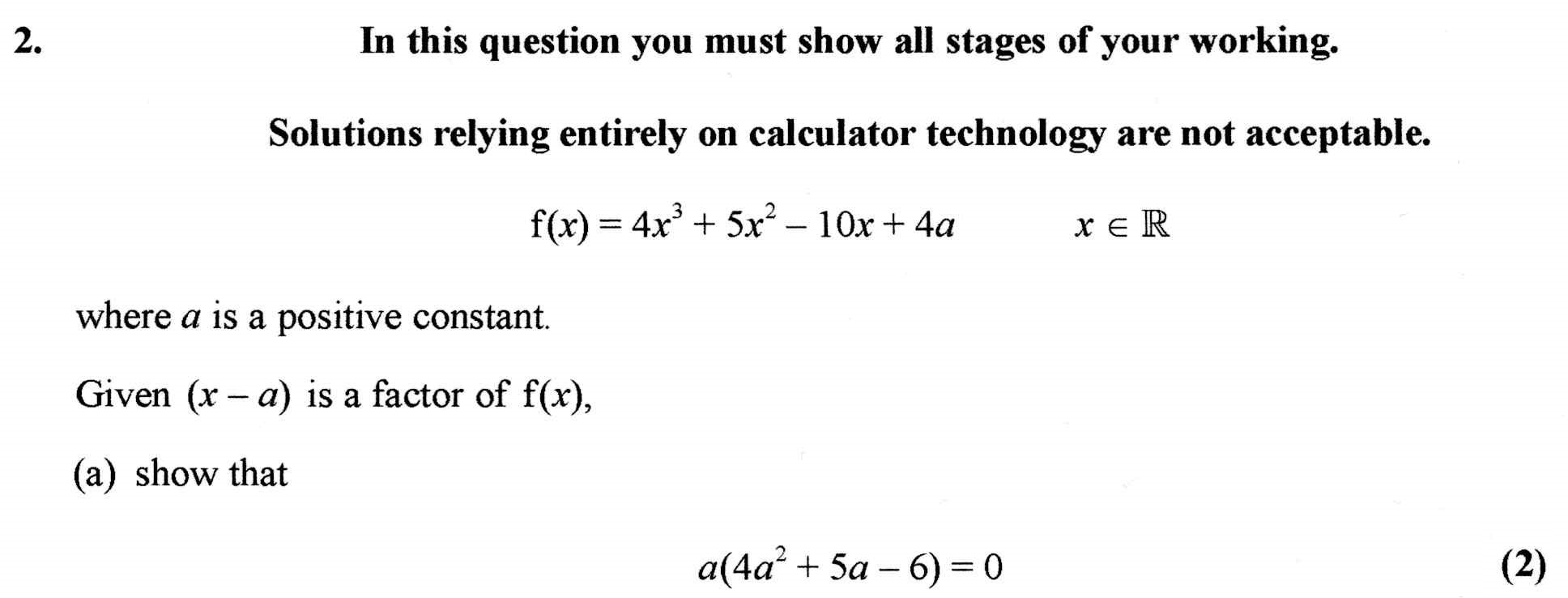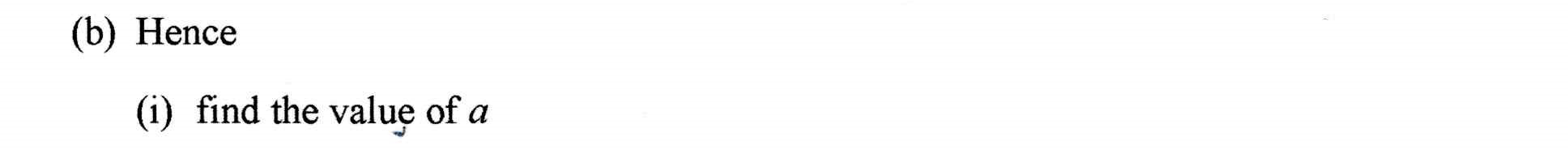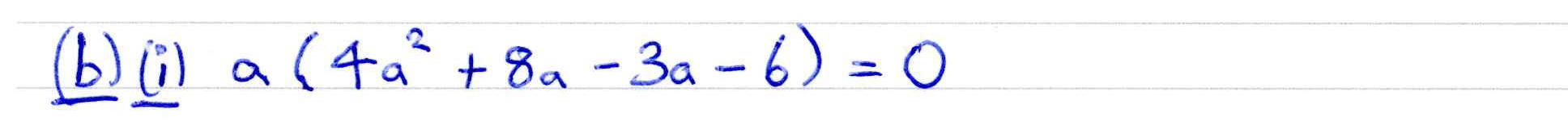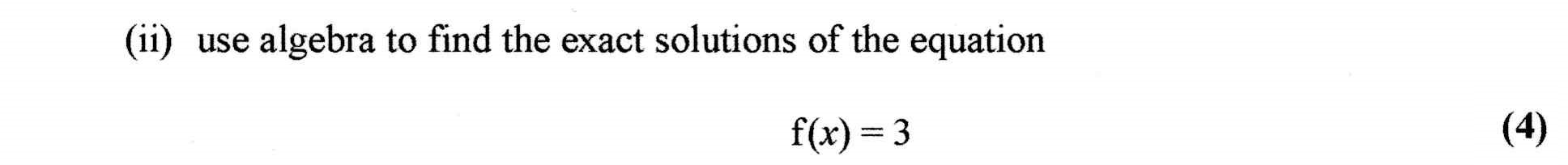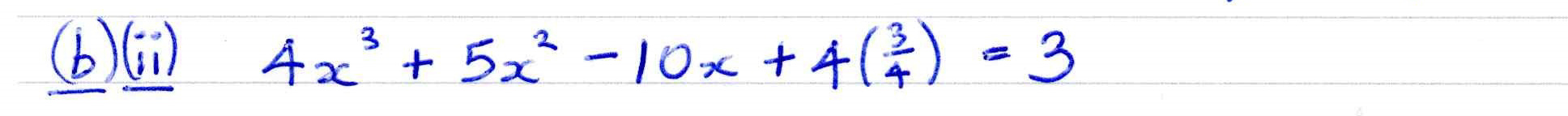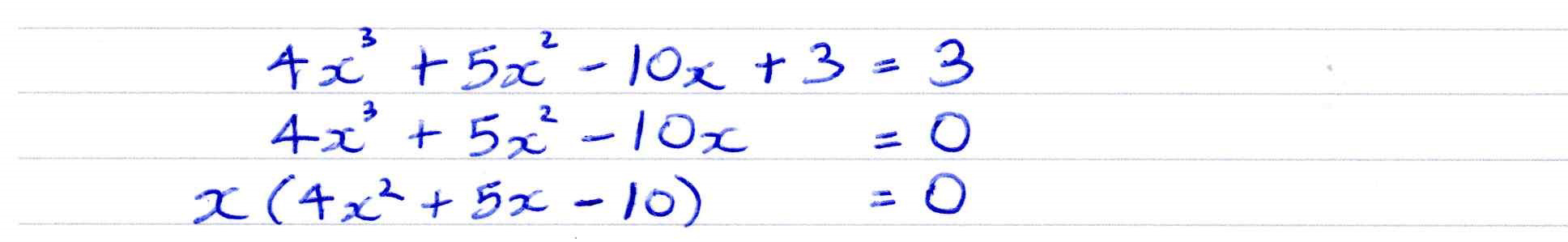##### Visualise Factor Theorem 1
The factor theorem states that:
If ${\color{blue} f(x) }$ is a polynomial and ${\color{blue} f(a) = 0 }$, then ${\color{blue} (x - a) }$ is a factor of ${\color{blue} f(x) }$.

This display helps show how this comes about.

You can drag the red point to help read coordinates.
##### Visualise Factor Theorem 2
The factor theorem also states that:
If ${\color{blue} f(x) }$ is a polynomial and ${\color{blue} f( {b \over a} ) = 0 }$, then ${\color{blue} (ax - b) }$ is a factor of ${\color{blue} f(x) }$.

This display helps show how this comes about.

You can drag the red point to help read coordinates.